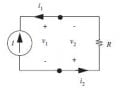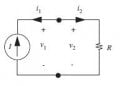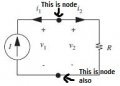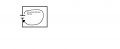# Simple Circuit Polarity Issues

#### stoltz1986

Joined Sep 21, 2015
7
Hello, I am studying the Foundations of Analog and Digital Circuits after going through the DC, some of the AC, and part of the Semiconductor texts on this website. I am having issues with understanding some of the polarity assignments that are made in some of the examples in this book.

There are two examples in the book, which states are, "physically the same".

Circuit #1Circuit #2In Circuit #1, i1 and i2 are flowing against the current source. Why are they pointing in the opposite direction of the current source?

I have two questions from these diagrams: (1) where did the opposing current i1 come from and (2) how can the polarity of a resistor be reversed to create an opposing voltage to that of the current source?

Thanks in advance and I apologize for the extremely simple questions. I know they're probably not very fun and/or challenging to answer.

#### StayatHomeElectronics

Joined Sep 25, 2008
1,073
The circuits #1 and #2 are the same but can be mathematically described in many different ways.

Many times when we analyze a circuit it is not quite as obvious which way the currents are flowing. We can define the current to go in any direction that we want. The resulting voltage polarities on the resistors must follow this definition. The current through resistor R flows from a more positive voltage to a more negative voltage in both figures. If we guess the polarity wrong on the current, the actual current will then just be negative.

Next you can apply other knowledge of electronics, like series circuits to help relate the current source current, I, to the other currents i1 and i2.

In circuit #1, I = -i1 = -i2. In circuit #2, I = -i1 = i2.

The same will happen with the voltages, if we defined, or guess, the current in the opposite direction of the actual current, the voltage will also be negative.

#### stoltz1986

Joined Sep 21, 2015
7
Ok. I think I get what you're saying, I just want to confirm. So these are pretty much examples of assigning direction of current or polarity in a sort of arbitrary manner and then after further analysis if the proposed direction of current is incorrect then it is negative? If correct, then positive?

#### StayatHomeElectronics

Joined Sep 25, 2008
1,073
From just looking at the two figures in your question, that is exactly the way I interpret it.

#### stoltz1986

Joined Sep 21, 2015
7

#### BramLabs

Joined Nov 21, 2013
98
From my point of view, i agree with the textbook.

If we remember the Kirchhoff Current Law : "At any node (junction) in an electrical circuit, the sum of currents flowing into that node is equal to the sum of currents flowing out of that node" ( Refference : https://en.wikipedia.org/wiki/Kirchhoff's_circuit_laws )From picture above, let's assume the total amount of current that leaving or going to the node is zero ( in this case based on the arrow of the current of I1 and I2, i assume the current is leaving FROM the node ). So we can say that : I1=-I2And from this picture above, we can see that the total amount of current leaving from the NODE is I1+I2=0

From both of the picture, we can also learn that the current flow based on the polarity of the voltage ( i mean is that THE CURRENT will flow from the positive side of voltage to the negative side of voltage )Oh yeah, i forgot to tell you, that basically, the node at the bottom of the circuit is the reference node.

Last edited: